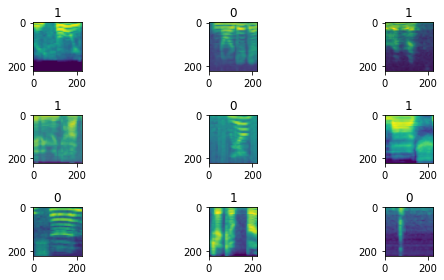# Runtime Error while running create_cnn

I am trying to build a image classifier on a bunch of mel spectogram images.

My image data is in two separate folders. I use the following code to load it in.

`tfms = get_transforms(do_flip=False, max_rotate=None, max_warp=None)`

`data = ImageDataBunch.from_df(data_path, test_df,valid_pct=0.2,ds_tfms = tfms, size = 224, bs = 16).normalize(imagenet_stats)`

Running the below code gives me this:

`data.show_batch(3, figsize=(8,4), hide_axis=False)`But when I run the following code:
`learn = create_cnn(data, models.resnet34, metrics=accuracy) learn.fit_one_cycle(4)`

I get this error:
`RuntimeError: Given groups=1, weight of size 64 1 7 7, expected input[16, 3, 224, 224] to have 1 channels, but got 3 channels instead`

Kindly help me with this problem or even pointers to where to look will be helpful. Thanks

Can you give some details about how you are defining your `tfms`?

I edited the original post. Here is the `tfms`

`tfms = get_transforms(do_flip=False, max_rotate=None, max_warp=None)`

Thank You